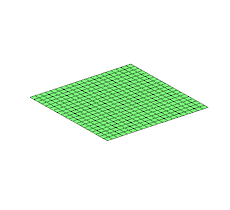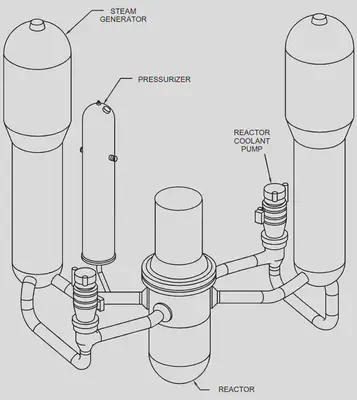# PDEs: what a nice hypercube you have here!

An hypercube is the generalisation of a square (2 dimensions) or a cube (3 dimensions) to any number of dimensions.

For the first post of the series about Partial Differential Equations (let’s call them PDEs from now on), I want to tell you about an ubiquitous issue in quantum computing: classical data transfer from and to a quantum computer.

“What’s the link with an hypercube ?” you might ask yourselves, well let me explain you.

## Partial differential equations in 20 seconds

A Partial Differential Equation (PDE) is an equation describing a certain quantity. For example, the heat equation that describes how heat spread over time or the wave equation that models how waves are propagating.Example of a 2-dimensional wave computed using the wave equation. Image from Wikipedia licensed under CC BY-SA 4.0 and modified to have a transparent background.

Independently of which PDE you consider, it will describe something, a quantity of interest. This something can be the temperature of a room, the amplitude of a wave, the speed of some particles, the weather, etc.

And this something does not live into nothing, it has to be part of a domain of definition.

## Domain of definition, or why we do not compute the universe each time

The domain of definition of the given quantity of interest is the space in which this quantity makes sense for our problem.

For the animated image representing a solution to the wave equation above (included below for convenience), the domain of definition is a 2-dimensional square.

The third dimension used in the animation is here to depict the value obtained by solving the wave equation, i.e., the wave amplitude.

Let’s say, for example, that you are interested in how the temperature of your bedroom evolves when you start the heater. In order to understand precisely its evolution, you can use the heat equation.

But does it makes sense to consider the temperature of everything around you in order to compute the temperature of your bedroom? Realistically, the temperature of this huge anticyclonic storm on Jupiter is not that important to know if you’re going to be cold in bed tonight.

So instead of computing the temperature of the whole universe, you only compute it on the domain of definition of your problem: your bedroom.

The most attentive among you might rightly ask themselves: “I agree about Jupiter, but there is surely a temperature difference in my bedroom between a cold winter day and an ongoing heatwave in summer, how do you account for that?”.

This will be the subject of the second post of this series, so for now let’s simplify and say that our room is completly isolated from the outside world.

## Hey! You! Quantum computer! Do you know that my bedroom is an hypercube?

We know that we only want to compute the temperature within the walls of our bedroom. But how do we tell the quantum computer about its limits? The domain of definition have to be communicated to the computer responsible for solving our equation.

And here comes our hypercube! One of the simplest domain of definition, the one that requires the least amount of information to be passed around, is the hypercube. “My bedroom is a cube (3-dimensional hypercube) with sides of 3 meters” is probably the simplest way to describe entirely a domain of definition.

But what if the architect that built your house was a tortured artist willing to “leave a trace of himself in this world” with your house? What if your bedroom floor was something like the following image?

Now, I challenge you to describe precisely this domain of definition (the bedroom) to someone in less than 10 seconds.

No seriously, stop reading for 10 seconds, and think about how you would describe this to someone.

What you just experienced is the first issue that quantum PDE solvers will have to overcome in order to hope solving PDEs in the future: how to communicate precisely and efficiently the domain of definition of our problem to the quantum computer?

A quantum PDE solver is the quantum algorithm used to solve the PDE we are interested in.

In our bedroom example, the quantum PDE solver is a quantum algorithm computing the solution of the heat equation.

And this is not an easy task, particularly for a quantum computer that is unable to efficiently read classical data.

## Wait, quantum computers cannot access data?

The question of inputing classical data (for example the description of a domain of definition) into a quantum computation is quite complex but here are some facts:

If the following points seems contradictory to you, re-read them attentively. They do not contradict each other.
1. You can load any classical data you want into a quantum computation.
2. For most of the classical data, loading it into a quantum computation is not efficient (i.e., has a cost that grows exponentially with the number of qubits used).
3. There are some kinds of classical data that can be efficiently loaded.
I will likely write one (or several) blog posts about the kinds of data that can be efficiently loaded. Stay tuned!
To find out more: have a look at the litterature about “Quantum Random Access Memory”, often abbreviated “QRAM”.

## So if my bedroom is not an hypercube, I won’t benefit from quantum computing?

No this is not what has been said. You might benefit from quantum computing even if your bedroom’s architect wanted you to suffer each and everyday of your life.

But whether or not using a quantum computer will be interesting (in terms of computational time) for a given bedroom shape is still an open question.

## Conclusion

In this post, I wanted to help you understand the first challenge that you might face when using quantum computers to solve real-world PDEs.

Just like the tortured-architect-bedroom I jokingly used in the previous sections, real-world domains of definitions are rarely hypercubes or easy to describe domains.

Think about a car, a combustion chamber, or the water circuits of a nuclear power plant (see image below). None of them are easy-to-describe domains of definition. None of them are simple and nice hypercubes.Example of a pressurized water reactor from the Nuclear Regulatory Commision (source).

## What’s next?

simplify and say that our room is completly isolated from the outside world.

This simplification is obviously wrong, as it leads to results that disagree with our observations, for example that the bedroom temperature is the same on a sunny day and during a cold winter.

In the next blog post, I will explore how we can consider the outside world, and why this consideration brings another issue on the table.

If you want to be notified when each post is out, you can add me on LinkedIn, I will do a LinkedIn post for each new blog post.

Also, if this post picked your interest or raised some questions in your mind, please feel free to send me a message 😉